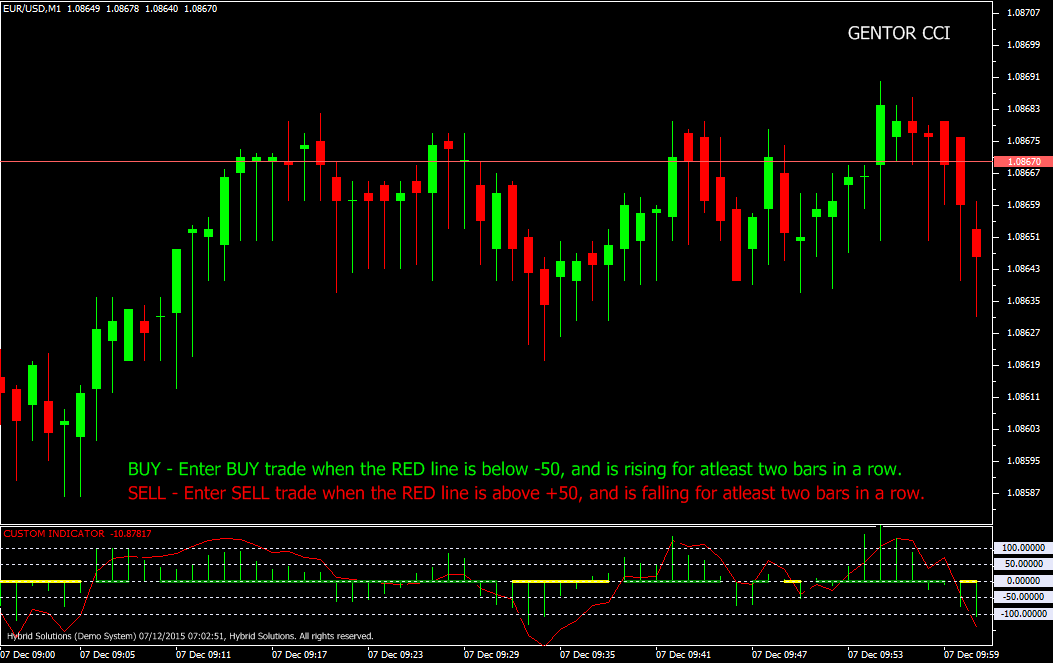# Gentor CCI

Free

Developed By: NeeduVerdeSolution

In stock

Gentor CCI is a powerful VertexFX client side VTL indicator that gives excellent entry and exit signals. This indicator is an enhancement of the Commodity Channel Index (CCI) indicator.

The CCI indicator provides us with hints when the market changes direction, and the strength of the trend in its running course. It comprises of two components, the fast CCI and the slow CCI. The fast CCI component which is the red line is the Commodity Channel Index calculated over the PERIOD_A bars – which is the shorter period. The slow CCI component, which is the green histogram is the Commodity Channel Index calculated over the PERIOD_B bars – which is the longer period.

SKU: 864 Categories: ,Gentor CCI is a powerful VertexFX client side VTL indicator that gives excellent entry and exit signals. This indicator is an enhancement of the Commodity Channel Index (CCI) indicator.

The CCI indicator provides us with hints when the market changes direction, and the strength of the trend in its running course. It comprises of two components, the fast CCI and the slow CCI. The fast CCI component which is the red line is the Commodity Channel Index calculated over the PERIOD_A bars – which is the shorter period. The slow CCI component, which is the green histogram is the Commodity Channel Index calculated over the PERIOD_B bars – which is the longer period.

The concept behind this indicator is that the faster CCI responds quickly to the change in trend direction compared to the slower CCI. When the faster CCI crosses above the slower CCI from below, it implies that a bullish trend is very likely. Similarly, when then faster CCI crosses below the slower CCI from above, it implies that a bearish trend is very likely.

Buy: Place Buy position when the Red line is below -50, and is rising for atleast two bars in a row.
Sell: Place Sell position when the Red line is above +50, and is falling for atleast two bars in a row.